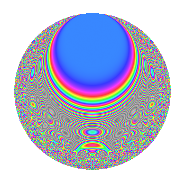Properties

 Label 2678.2.aLevel 2678 Weight 2 Character orbit a Rep. character $$\chi_{2678}(1,\cdot)$$ Character field $$\Q$$ Dimension 101 Newforms 23 Sturm bound 728 Trace bound 7

Related objects

Defining parameters

 Level: $$N$$ = $$2678 = 2 \cdot 13 \cdot 103$$ Weight: $$k$$ = $$2$$ Character orbit: $$[\chi]$$ = 2678.a (trivial) Character field: $$\Q$$ Newforms: $$23$$ Sturm bound: $$728$$ Trace bound: $$7$$ Distinguishing $$T_p$$: $$3$$, $$5$$, $$7$$

Dimensions

The following table gives the dimensions of various subspaces of $$M_{2}(\Gamma_0(2678))$$.

Total New Old
Modular forms 368 101 267
Cusp forms 361 101 260
Eisenstein series 7 0 7

The following table gives the dimensions of the cuspidal new subspaces with specified eigenvalues for the Atkin-Lehner operators and the Fricke involution.

$$2$$$$13$$$$103$$FrickeDim.
$$+$$$$+$$$$+$$$$+$$$$14$$
$$+$$$$+$$$$-$$$$-$$$$12$$
$$+$$$$-$$$$+$$$$-$$$$16$$
$$+$$$$-$$$$-$$$$+$$$$8$$
$$-$$$$+$$$$+$$$$-$$$$13$$
$$-$$$$+$$$$-$$$$+$$$$11$$
$$-$$$$-$$$$+$$$$+$$$$8$$
$$-$$$$-$$$$-$$$$-$$$$19$$
Plus space$$+$$$$41$$
Minus space$$-$$$$60$$

Trace form

 $$101q$$ $$\mathstrut +\mathstrut q^{2}$$ $$\mathstrut +\mathstrut 101q^{4}$$ $$\mathstrut +\mathstrut 2q^{5}$$ $$\mathstrut +\mathstrut q^{8}$$ $$\mathstrut +\mathstrut 93q^{9}$$ $$\mathstrut +\mathstrut O(q^{10})$$ $$101q$$ $$\mathstrut +\mathstrut q^{2}$$ $$\mathstrut +\mathstrut 101q^{4}$$ $$\mathstrut +\mathstrut 2q^{5}$$ $$\mathstrut +\mathstrut q^{8}$$ $$\mathstrut +\mathstrut 93q^{9}$$ $$\mathstrut -\mathstrut 6q^{10}$$ $$\mathstrut +\mathstrut q^{13}$$ $$\mathstrut -\mathstrut 4q^{14}$$ $$\mathstrut +\mathstrut 101q^{16}$$ $$\mathstrut +\mathstrut 22q^{17}$$ $$\mathstrut -\mathstrut 3q^{18}$$ $$\mathstrut -\mathstrut 4q^{19}$$ $$\mathstrut +\mathstrut 2q^{20}$$ $$\mathstrut +\mathstrut 16q^{21}$$ $$\mathstrut +\mathstrut 4q^{22}$$ $$\mathstrut +\mathstrut 16q^{23}$$ $$\mathstrut +\mathstrut 87q^{25}$$ $$\mathstrut +\mathstrut 5q^{26}$$ $$\mathstrut +\mathstrut 36q^{27}$$ $$\mathstrut -\mathstrut 18q^{29}$$ $$\mathstrut -\mathstrut 12q^{30}$$ $$\mathstrut -\mathstrut 32q^{31}$$ $$\mathstrut +\mathstrut q^{32}$$ $$\mathstrut -\mathstrut 8q^{33}$$ $$\mathstrut +\mathstrut 18q^{34}$$ $$\mathstrut +\mathstrut 28q^{35}$$ $$\mathstrut +\mathstrut 93q^{36}$$ $$\mathstrut -\mathstrut 22q^{37}$$ $$\mathstrut +\mathstrut 4q^{38}$$ $$\mathstrut -\mathstrut 4q^{39}$$ $$\mathstrut -\mathstrut 6q^{40}$$ $$\mathstrut +\mathstrut 10q^{41}$$ $$\mathstrut +\mathstrut 28q^{42}$$ $$\mathstrut +\mathstrut 40q^{43}$$ $$\mathstrut +\mathstrut 18q^{45}$$ $$\mathstrut -\mathstrut 16q^{46}$$ $$\mathstrut -\mathstrut 16q^{47}$$ $$\mathstrut +\mathstrut 121q^{49}$$ $$\mathstrut +\mathstrut 47q^{50}$$ $$\mathstrut +\mathstrut 44q^{51}$$ $$\mathstrut +\mathstrut q^{52}$$ $$\mathstrut -\mathstrut 2q^{53}$$ $$\mathstrut +\mathstrut 40q^{55}$$ $$\mathstrut -\mathstrut 4q^{56}$$ $$\mathstrut +\mathstrut 48q^{57}$$ $$\mathstrut -\mathstrut 10q^{58}$$ $$\mathstrut +\mathstrut 4q^{59}$$ $$\mathstrut +\mathstrut 6q^{61}$$ $$\mathstrut +\mathstrut 8q^{62}$$ $$\mathstrut +\mathstrut 16q^{63}$$ $$\mathstrut +\mathstrut 101q^{64}$$ $$\mathstrut +\mathstrut 2q^{65}$$ $$\mathstrut +\mathstrut 16q^{66}$$ $$\mathstrut +\mathstrut 22q^{68}$$ $$\mathstrut -\mathstrut 48q^{69}$$ $$\mathstrut -\mathstrut 32q^{70}$$ $$\mathstrut +\mathstrut 8q^{71}$$ $$\mathstrut -\mathstrut 3q^{72}$$ $$\mathstrut -\mathstrut 6q^{73}$$ $$\mathstrut -\mathstrut 30q^{74}$$ $$\mathstrut +\mathstrut 4q^{75}$$ $$\mathstrut -\mathstrut 4q^{76}$$ $$\mathstrut +\mathstrut 16q^{77}$$ $$\mathstrut -\mathstrut 4q^{78}$$ $$\mathstrut -\mathstrut 40q^{79}$$ $$\mathstrut +\mathstrut 2q^{80}$$ $$\mathstrut +\mathstrut 93q^{81}$$ $$\mathstrut +\mathstrut 2q^{82}$$ $$\mathstrut +\mathstrut 4q^{83}$$ $$\mathstrut +\mathstrut 16q^{84}$$ $$\mathstrut -\mathstrut 28q^{85}$$ $$\mathstrut -\mathstrut 16q^{86}$$ $$\mathstrut +\mathstrut 8q^{87}$$ $$\mathstrut +\mathstrut 4q^{88}$$ $$\mathstrut -\mathstrut 86q^{89}$$ $$\mathstrut -\mathstrut 34q^{90}$$ $$\mathstrut +\mathstrut 12q^{91}$$ $$\mathstrut +\mathstrut 16q^{92}$$ $$\mathstrut -\mathstrut 32q^{93}$$ $$\mathstrut -\mathstrut 20q^{94}$$ $$\mathstrut -\mathstrut 24q^{95}$$ $$\mathstrut +\mathstrut 10q^{97}$$ $$\mathstrut -\mathstrut 7q^{98}$$ $$\mathstrut -\mathstrut 16q^{99}$$ $$\mathstrut +\mathstrut O(q^{100})$$

Decomposition of $$S_{2}^{\mathrm{new}}(\Gamma_0(2678))$$ into irreducible Hecke orbits

Label Dim. $$A$$ Field CM Traces A-L signs $q$-expansion
$$a_2$$ $$a_3$$ $$a_5$$ $$a_7$$ 2 13 103
2678.2.a.a $$1$$ $$21.384$$ $$\Q$$ None $$-1$$ $$-2$$ $$-2$$ $$-1$$ $$+$$ $$+$$ $$-$$ $$q-q^{2}-2q^{3}+q^{4}-2q^{5}+2q^{6}-q^{7}+\cdots$$
2678.2.a.b $$1$$ $$21.384$$ $$\Q$$ None $$-1$$ $$-2$$ $$0$$ $$-1$$ $$+$$ $$-$$ $$-$$ $$q-q^{2}-2q^{3}+q^{4}+2q^{6}-q^{7}-q^{8}+\cdots$$
2678.2.a.c $$1$$ $$21.384$$ $$\Q$$ None $$-1$$ $$-1$$ $$-3$$ $$-3$$ $$+$$ $$-$$ $$-$$ $$q-q^{2}-q^{3}+q^{4}-3q^{5}+q^{6}-3q^{7}+\cdots$$
2678.2.a.d $$1$$ $$21.384$$ $$\Q$$ None $$-1$$ $$-1$$ $$3$$ $$0$$ $$+$$ $$-$$ $$-$$ $$q-q^{2}-q^{3}+q^{4}+3q^{5}+q^{6}-q^{8}+\cdots$$
2678.2.a.e $$1$$ $$21.384$$ $$\Q$$ None $$-1$$ $$2$$ $$-2$$ $$3$$ $$+$$ $$+$$ $$-$$ $$q-q^{2}+2q^{3}+q^{4}-2q^{5}-2q^{6}+3q^{7}+\cdots$$
2678.2.a.f $$1$$ $$21.384$$ $$\Q$$ None $$-1$$ $$2$$ $$0$$ $$-5$$ $$+$$ $$-$$ $$-$$ $$q-q^{2}+2q^{3}+q^{4}-2q^{6}-5q^{7}-q^{8}+\cdots$$
2678.2.a.g $$1$$ $$21.384$$ $$\Q$$ None $$-1$$ $$2$$ $$0$$ $$0$$ $$+$$ $$-$$ $$-$$ $$q-q^{2}+2q^{3}+q^{4}-2q^{6}-q^{8}+q^{9}+\cdots$$
2678.2.a.h $$1$$ $$21.384$$ $$\Q$$ None $$-1$$ $$3$$ $$1$$ $$5$$ $$+$$ $$-$$ $$+$$ $$q-q^{2}+3q^{3}+q^{4}+q^{5}-3q^{6}+5q^{7}+\cdots$$
2678.2.a.i $$1$$ $$21.384$$ $$\Q$$ None $$1$$ $$-2$$ $$-4$$ $$-3$$ $$-$$ $$+$$ $$+$$ $$q+q^{2}-2q^{3}+q^{4}-4q^{5}-2q^{6}-3q^{7}+\cdots$$
2678.2.a.j $$1$$ $$21.384$$ $$\Q$$ None $$1$$ $$-2$$ $$2$$ $$-3$$ $$-$$ $$-$$ $$+$$ $$q+q^{2}-2q^{3}+q^{4}+2q^{5}-2q^{6}-3q^{7}+\cdots$$
2678.2.a.k $$1$$ $$21.384$$ $$\Q$$ None $$1$$ $$0$$ $$-2$$ $$-1$$ $$-$$ $$-$$ $$+$$ $$q+q^{2}+q^{4}-2q^{5}-q^{7}+q^{8}-3q^{9}+\cdots$$
2678.2.a.l $$1$$ $$21.384$$ $$\Q$$ None $$1$$ $$1$$ $$-1$$ $$0$$ $$-$$ $$-$$ $$+$$ $$q+q^{2}+q^{3}+q^{4}-q^{5}+q^{6}+q^{8}+\cdots$$
2678.2.a.m $$1$$ $$21.384$$ $$\Q$$ None $$1$$ $$1$$ $$1$$ $$-4$$ $$-$$ $$+$$ $$-$$ $$q+q^{2}+q^{3}+q^{4}+q^{5}+q^{6}-4q^{7}+\cdots$$
2678.2.a.n $$1$$ $$21.384$$ $$\Q$$ None $$1$$ $$3$$ $$-1$$ $$4$$ $$-$$ $$+$$ $$+$$ $$q+q^{2}+3q^{3}+q^{4}-q^{5}+3q^{6}+4q^{7}+\cdots$$
2678.2.a.o $$2$$ $$21.384$$ $$\Q(\sqrt{5})$$ None $$2$$ $$-3$$ $$-2$$ $$0$$ $$-$$ $$-$$ $$+$$ $$q+q^{2}+(-1-\beta )q^{3}+q^{4}-2\beta q^{5}+\cdots$$
2678.2.a.p $$3$$ $$21.384$$ 3.3.621.1 None $$-3$$ $$-3$$ $$0$$ $$3$$ $$+$$ $$-$$ $$-$$ $$q-q^{2}+(-1+\beta _{1})q^{3}+q^{4}+(1-\beta _{1}+\cdots)q^{6}+\cdots$$
2678.2.a.q $$3$$ $$21.384$$ 3.3.316.1 None $$3$$ $$-4$$ $$0$$ $$-5$$ $$-$$ $$-$$ $$+$$ $$q+q^{2}+(-1-\beta _{1})q^{3}+q^{4}+\beta _{2}q^{5}+\cdots$$
2678.2.a.r $$10$$ $$21.384$$ $$\mathbb{Q}[x]/(x^{10} - \cdots)$$ None $$-10$$ $$0$$ $$9$$ $$9$$ $$+$$ $$+$$ $$-$$ $$q-q^{2}+\beta _{1}q^{3}+q^{4}+(1-\beta _{3})q^{5}-\beta _{1}q^{6}+\cdots$$
2678.2.a.s $$10$$ $$21.384$$ $$\mathbb{Q}[x]/(x^{10} - \cdots)$$ None $$10$$ $$-6$$ $$-3$$ $$-10$$ $$-$$ $$+$$ $$-$$ $$q+q^{2}+(-1+\beta _{1})q^{3}+q^{4}-\beta _{5}q^{5}+\cdots$$
2678.2.a.t $$11$$ $$21.384$$ $$\mathbb{Q}[x]/(x^{11} - \cdots)$$ None $$11$$ $$6$$ $$7$$ $$7$$ $$-$$ $$+$$ $$+$$ $$q+q^{2}+(1-\beta _{1})q^{3}+q^{4}+(1+\beta _{5})q^{5}+\cdots$$
2678.2.a.u $$14$$ $$21.384$$ $$\mathbb{Q}[x]/(x^{14} - \cdots)$$ None $$-14$$ $$0$$ $$-5$$ $$-11$$ $$+$$ $$+$$ $$+$$ $$q-q^{2}+\beta _{1}q^{3}+q^{4}-\beta _{3}q^{5}-\beta _{1}q^{6}+\cdots$$
2678.2.a.v $$15$$ $$21.384$$ $$\mathbb{Q}[x]/(x^{15} - \cdots)$$ None $$-15$$ $$0$$ $$3$$ $$3$$ $$+$$ $$-$$ $$+$$ $$q-q^{2}+\beta _{1}q^{3}+q^{4}+\beta _{6}q^{5}-\beta _{1}q^{6}+\cdots$$
2678.2.a.w $$19$$ $$21.384$$ $$\mathbb{Q}[x]/(x^{19} - \cdots)$$ None $$19$$ $$6$$ $$1$$ $$13$$ $$-$$ $$-$$ $$-$$ $$q+q^{2}+\beta _{1}q^{3}+q^{4}+\beta _{8}q^{5}+\beta _{1}q^{6}+\cdots$$

Decomposition of $$S_{2}^{\mathrm{old}}(\Gamma_0(2678))$$ into lower level spaces

$$S_{2}^{\mathrm{old}}(\Gamma_0(2678)) \cong$$ $$S_{2}^{\mathrm{new}}(\Gamma_0(26))$$$$^{\oplus 2}$$$$\oplus$$$$S_{2}^{\mathrm{new}}(\Gamma_0(103))$$$$^{\oplus 4}$$$$\oplus$$$$S_{2}^{\mathrm{new}}(\Gamma_0(206))$$$$^{\oplus 2}$$$$\oplus$$$$S_{2}^{\mathrm{new}}(\Gamma_0(1339))$$$$^{\oplus 2}$$